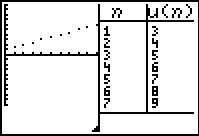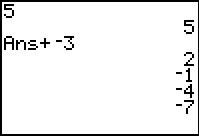# Activities

••• ##### Subject Area

• Math: Algebra II: Sequences
• Math: Algebra II: Series

• ##### Author9-12

15 Minutes

• ##### Device
• TI-83 Plus Family
• TI-84 Plus
• TI-84 Plus Silver Edition
•TI-84 Plus C Silver Edition
•TI-84 Plus CE
• ##### Report an Issue

Sequence Investigation#### Activity Overview

Students use the calculator to create an arithmetic sequence and explore the effect of each variable in the formula of the nth term of an arithmetic sequence.

#### Key Steps

•Students will use the calculator to create an arithmetic sequence and determine the formula for finding the nth term in the sequence.

•Then students will explore the effects of the initial term and the common difference on the table of sequence values and the graph.